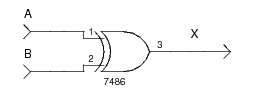# XOR Gate

An XOR (exclusive OR) gate is a fundamental binary logic gate with two inputs and one output. The output is `1` if only one input is `1`. An XOR gate with more than two inputs gives an output of `1` if an odd number of inputs is `1`. Some authors call the XOR gate with N inputs the odd function.

The following truth table gives the specification for a two input XOR gate with output `X`:

` A `` B `` X `
`0``0``0`
`0``1``1`
`1``0``1`
`1``1``0`

The schematic form of the XOR gate is:Below is a Verilog structural model for the XOR gate. In the code, the first argument to `xor` is the gate output, the other arguments are inputs.

```	    ```
module xor_gate(X, A, B);
output X;
input  A;
input  B;

xor(X, A, B);
endmodule
```
```

## References

Mano, M. Morris, and Kime, Charles R. Logic and Computer Design Fundamentals. 2nd Edition. Prentice Hall, 2000.
Kleitz, W. Digital Microprocessor Fundamentals. 3rd Edition. Prentice Hall, 2000.